# Perron-Frobenius theorem

(Redirected from Perron–Frobenius theorem)

Let a real square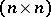-matrixbe considered as an operator on, let it be without invariant coordinate subspaces (such a matrix is called indecomposable) and let it be non-negative (i.e. all its elements are non-negative). Also, let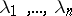be its eigen values, enumerated such thatThen,

1) the number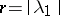is a simple positive root of the characteristic polynomial of;

2) there exists an eigen vector ofwith positive coordinates corresponding to;

3) the numberscoincide, apart from their numbering, with the numbers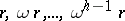, where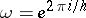;

4) the product of any eigen value ofbyis an eigen value of;

5) forone can find a permutation of the rows and columns that reducesto the formwhere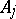is a matrix of order.

O. Perron proved the assertions 1) and 2) for positive matrices in , while G. Frobenius  gave the full form of the theorem.